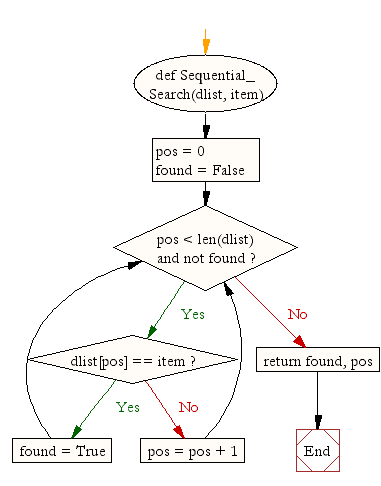## ~ Searching Algorithms - Linear or Sequential Search

Searching Algorithms in Python

### Linear or Sequential Search

In computer science, linear search or sequential search is a method for finding a target value within a list. It sequentially checks each element of the list for the target value until a match is found or until all the elements have been searched.

Linear search runs in at worst linear time and makes at most n comparisons, where n is the length of the list. If each element is equally likely to be searched, then linear search has an average case of n/2 comparisons, but the average case can be affected if the search probabilities for each element vary. Linear search is rarely practical because other search algorithms and schemes, such as the binary search algorithm and hash tables, allow significantly faster searching for all but short lists

### How to code a Linear search

A step by step implementation of coding a linear search in python

### Challenge

#1 Write a Python program to search using the sequential/linear search method

#2 Comment each line of the python solution below to show your understanding of the algorithm

### Solution

def Sequential_Search(dlist, item):

pos = 0
found = False

if dlist[pos] == item:
found = True
else:
pos = pos + 1

return found, pos

print(Sequential_Search([11,23,58,31,56,77,43,12,65,19],31))



### Flowchart### In pseudocode this would look like:

OUTPUT "Which customer number would you like to look up?"
INPUT user inputs customer number
STORE the user's input in the customer_number variable
counter = 1
(we need to count the number of records that we have searched through)
more_records = True
(we need a flag to say if more records are available to search through
or if we have reached the end of the database)
WHILE more_records = True:
IF counter = customer_number THEN
Exit the loop
ELSE
add 1 to counter

Linear search sequentially checks each element of the list until it finds an element that matches the target value. If the algorithm reaches the end of the list, the search terminates unsuccessfully.

### Basic algorithm

Given a list L of n elements with values or records L0 ... Ln−1, and target value T, the following subroutine uses linear search to find the index of the target T in L.

1. Set i to 0.
2. If Li = T, the search terminates successfully; return i.
3. Increase i by 1.
4. If i < n, go to step 2. Otherwise, the search terminates unsuccessfully.

### With a sentinel

The basic algorithm above makes two comparisons per iteration: one to check if Li equals t, and the other to check if i still points to a valid index of the list. By adding an extra record Ln to the list (a sentinel value) that equals the target, the second comparison can be eliminated until the end of the search, making the algorithm faster. The search will reach the sentinel if the target is not contained within the list.

1. Set i to 0.
2. If Li = T, go to step 4.
3. Increase i by 1 and go to step 2.
4. If i < n, the search terminates successfully; return i. Else, the search terminates unsuccessfully.

### In an ordered table

If the list is ordered such that L0 ≤ L1 ... ≤ Ln−1, the search can establish the absence of the target more quickly by concluding the search once Li exceeds the target. This variation requires a sentinel that is greater than the target.

1. Set i to 0.
2. If Li ≥ T, or i ≥ n, go to step 4.
3. Increase i by 1 and go to step 2.
4. If Li = T, the search terminates successfully; return i. Else, the search terminates unsuccessfully.

## Analysis

For a list with n items, the best case is when the value is equal to the first element of the list, in which case only one comparison is needed. The worst case is when the value is not in the list (or occurs only once at the end of the list), in which case n comparisons are needed.

If the value being sought occurs k times in the list, and all orderings of the list are equally likely, the expected number of comparisons isFor example, if the value being sought occurs once in the list, and all orderings of the list are equally likely, the expected number of comparisons is. However, if it is known that it occurs once, then at most n - 1 comparisons are needed, and the expected number of comparisons is(for example, for n = 2 this is 1, corresponding to a single if-then-else construct).

Either way, asymptotically the worst-case cost and the expected cost of linear search are both O(n).

### Non-uniform probabilities

The performance of linear search improves if the desired value is more likely to be near the beginning of the list than to its end. Therefore, if some values are much more likely to be searched than others, it is desirable to place them at the beginning of the list.

In particular, when the list items are arranged in order of decreasing probability, and these probabilities are geometrically distributed, the cost of linear search is only O(1). If the table size n is large enough, linear search will be faster than binary search, whose cost is O(log n).

## Application

Linear search is usually very simple to implement, and is practical when the list has only a few elements, or when performing a single search in an unordered list.

When many values have to be searched in the same list, it often pays to pre-process the list in order to use a faster method. For example, one may sort the list and use binary search, or build an efficient search data structure from it. Should the content of the list change frequently, repeated re-organization may be more trouble than it is worth.

As a result, even though in theory other search algorithms may be faster than linear search (for instance binary search), in practice even on medium-sized arrays (around 100 items or less) it might be infeasible to use anything else. On larger arrays, it only makes sense to use other, faster search methods if the data is large enough, because the initial time to prepare (sort) the data is comparable to many linear searches